Learn about smartphone sensors in the next Xcel masterclass with Tinkerbee CEO, Anupam! Learn about smartphone sensors in the next Xcel masterclass with Tinkerbee CEO, Anupam!

# Kerala Plus Two Maths Previous Question Papers and Answers 2015 In PDF

## Kerala Class 12th Maths Question Paper 2015 Solved – Free Download

Kerala Plus Two (Class 12) maths 2015 question paper with answers are provided here in a downloadable pdf format and also in the text, so that the students can obtain them which gives a boost to their preparation. Along with the solutions, maths question paper 2015 Class 12 is also made available for reference. 12th Students are able to access all the Kerala board previous year maths question papers. The solutions provided by BYJU’S are prepared by industry experts and can be relied upon for guidance. Also, it will assist the students in analyzing their difficult areas. The students can use 2015 Maths question papers and also other previous year papers for regular practice sessions.

### KBPE Plus Two (Class 12th) Maths Question Paper With Solution 2015

#### QUESTION PAPER CODE 7018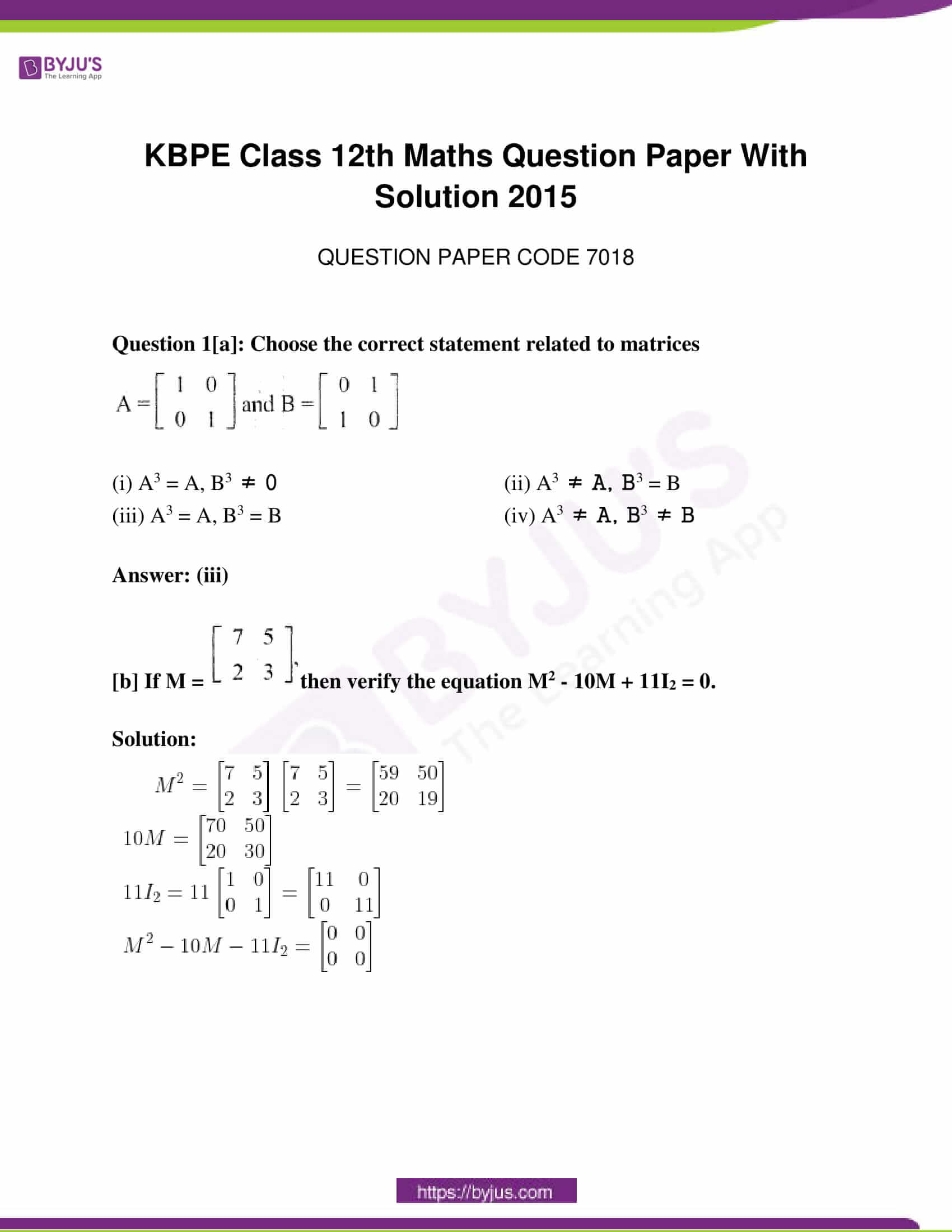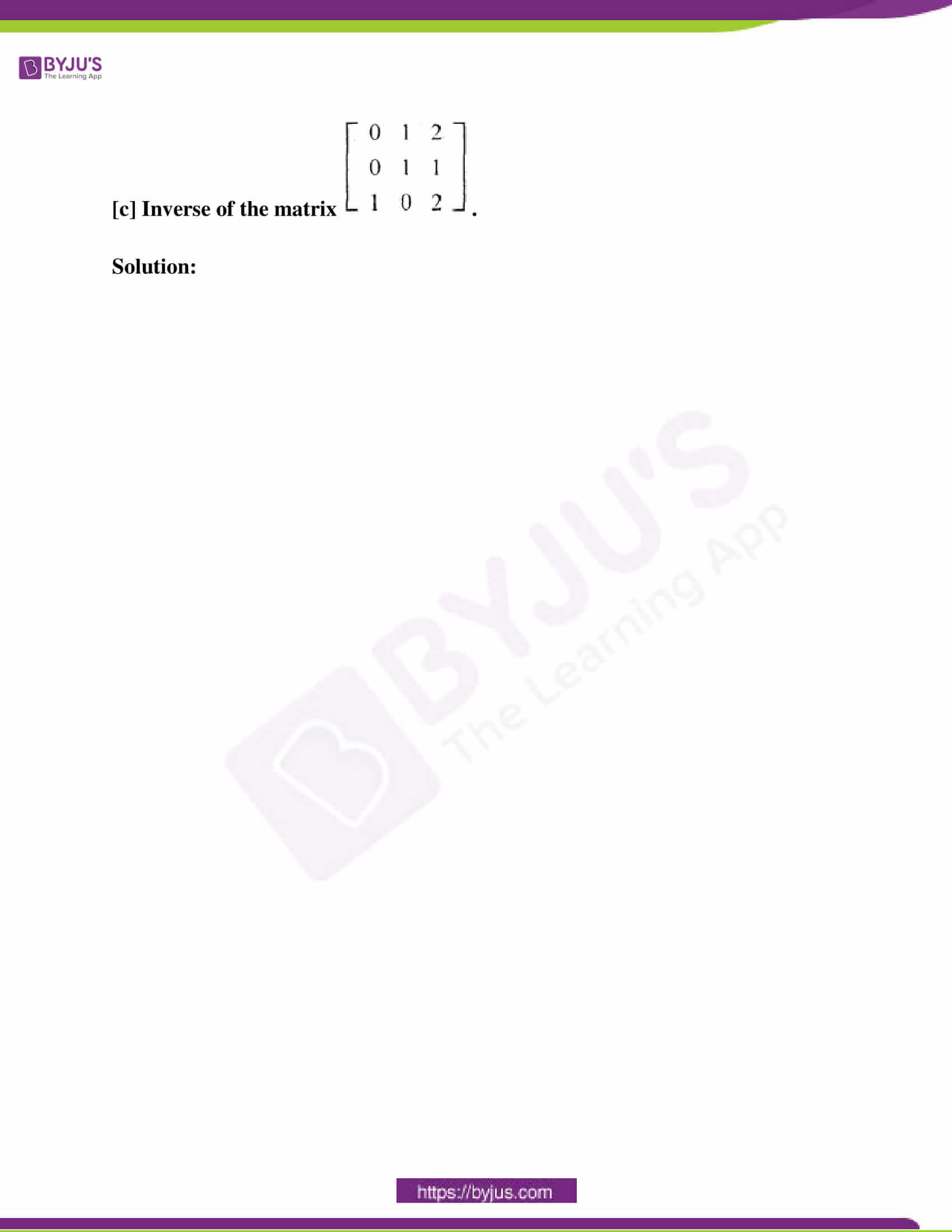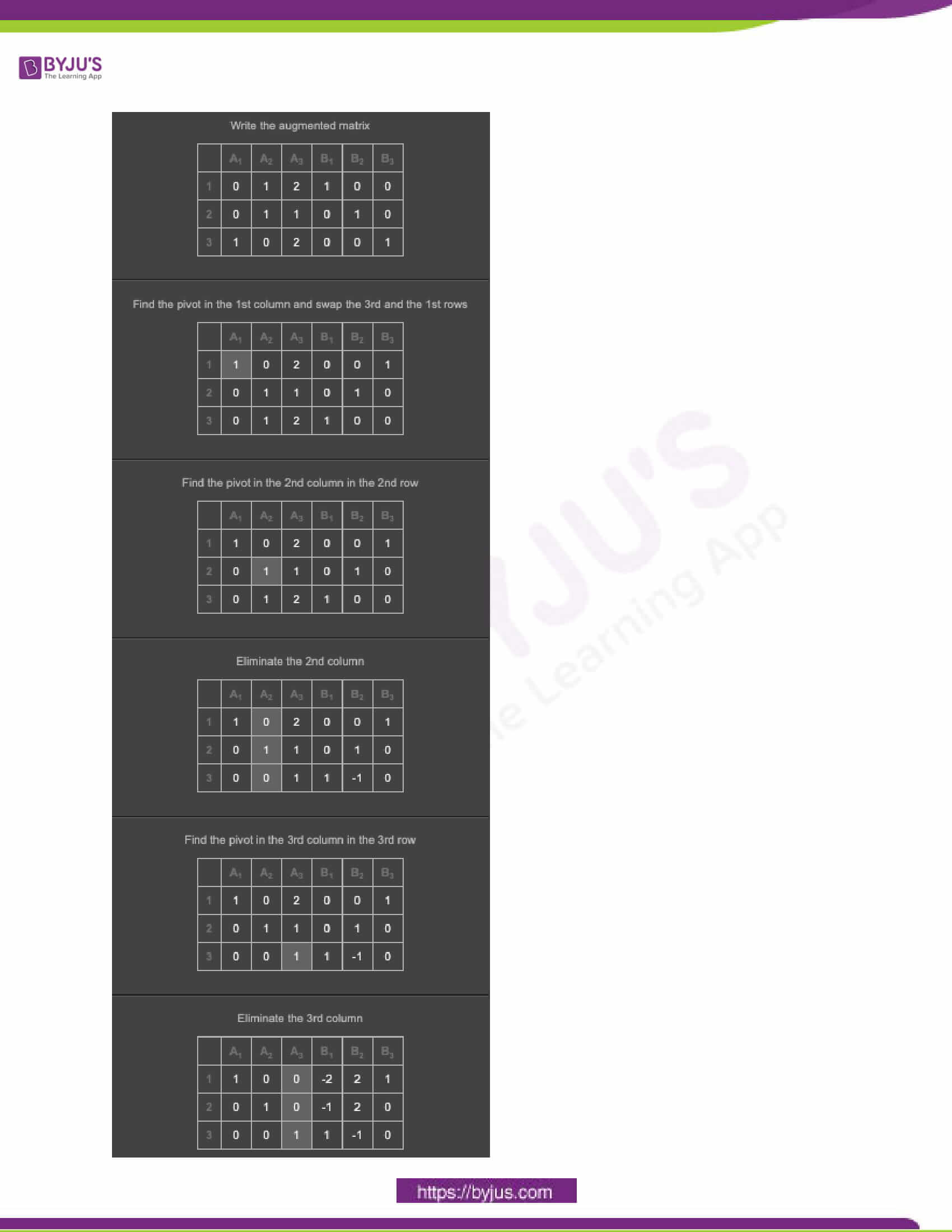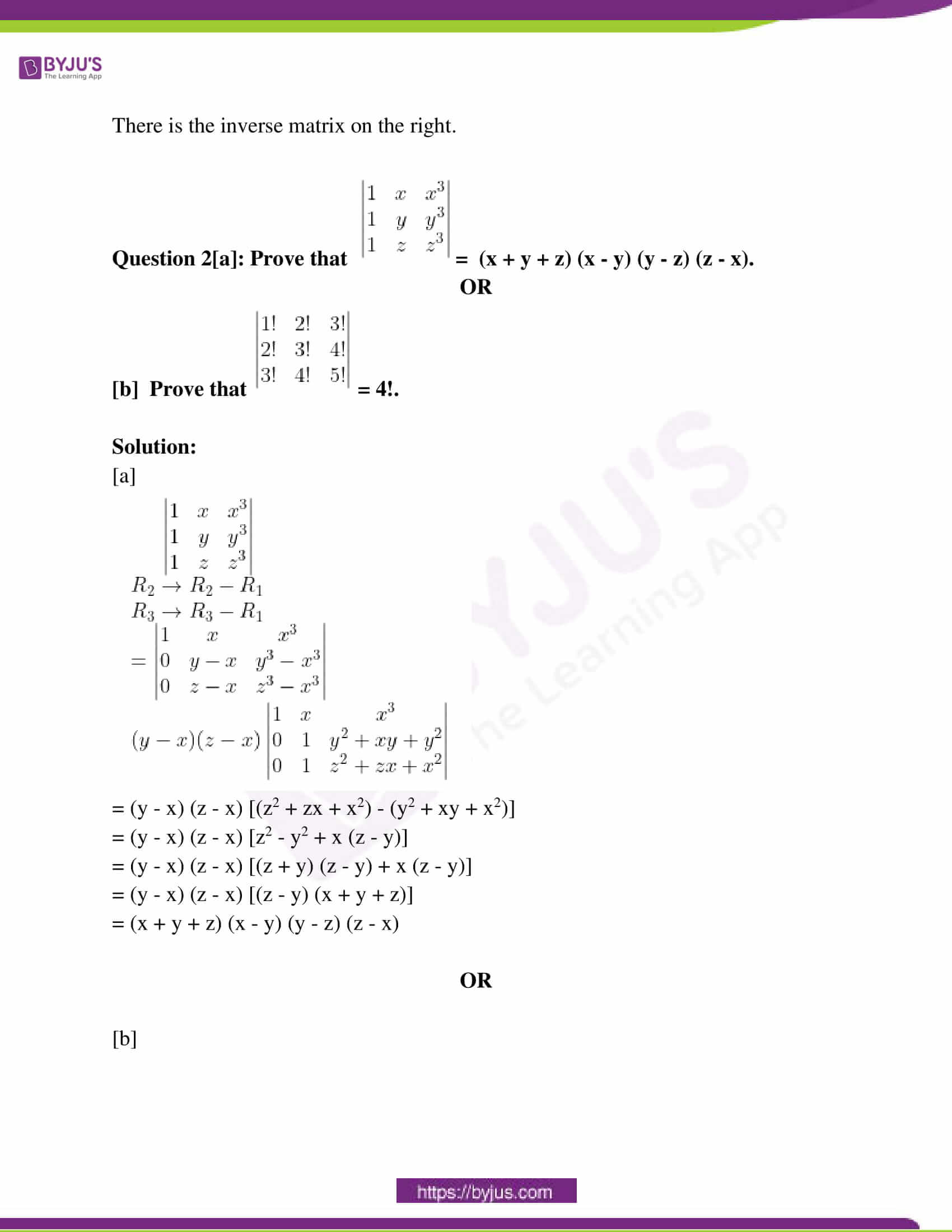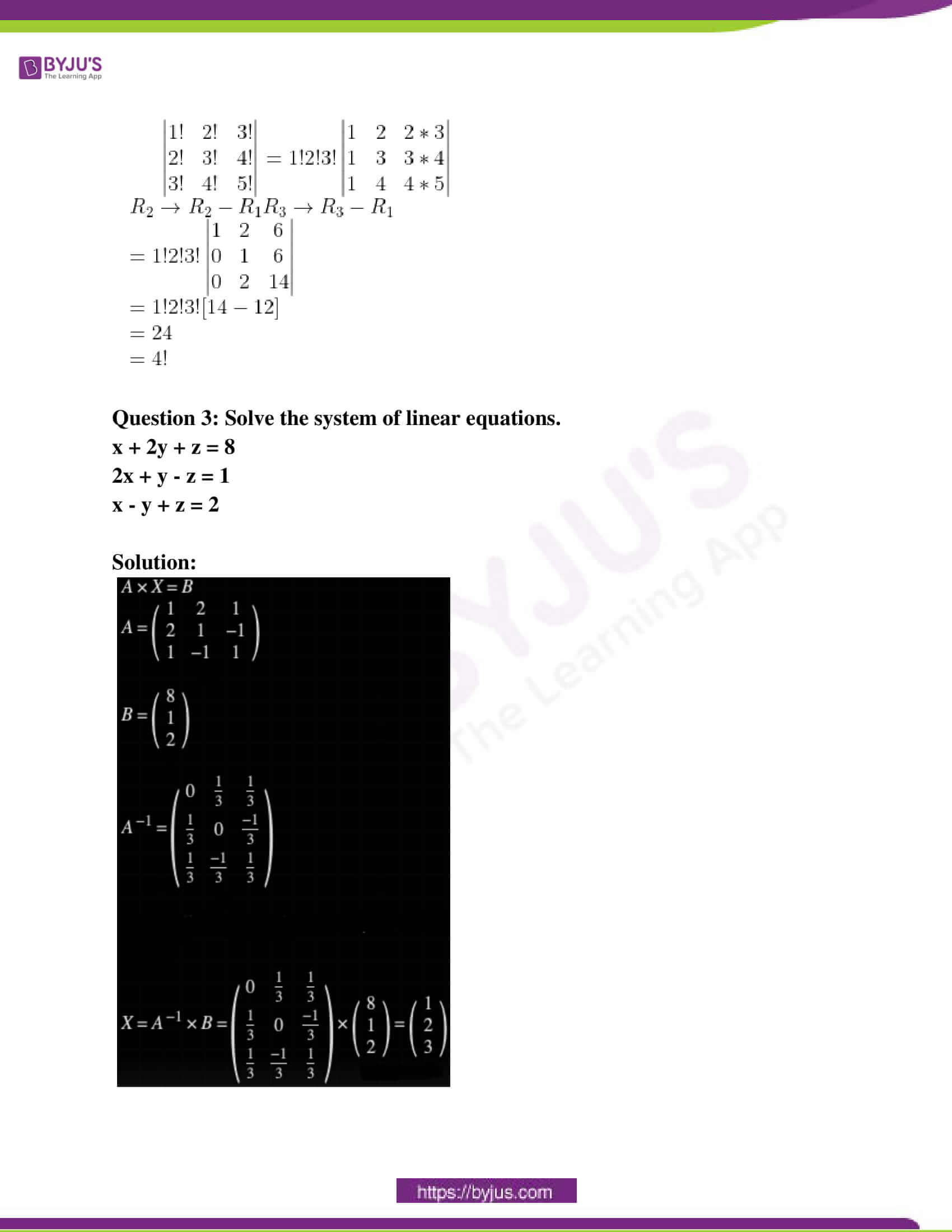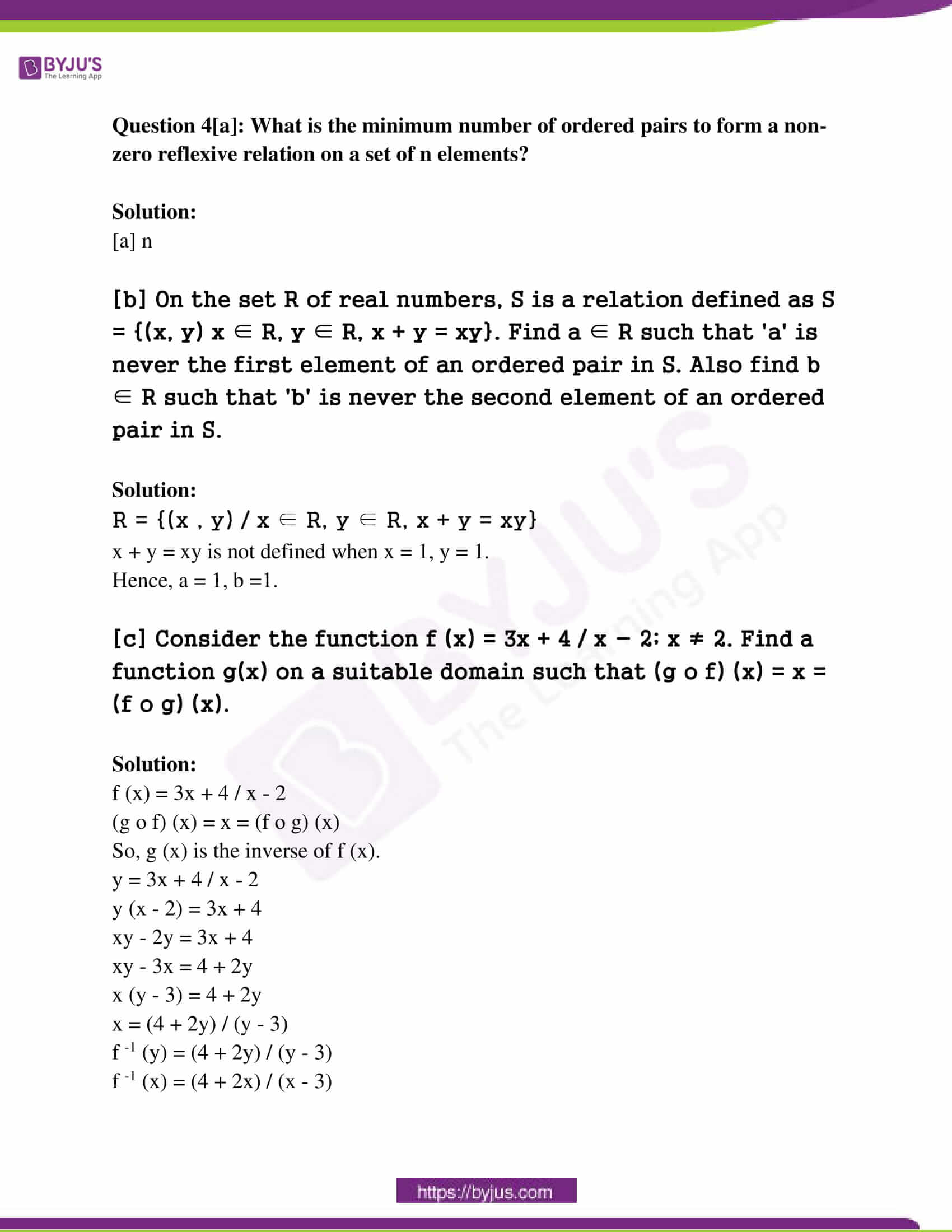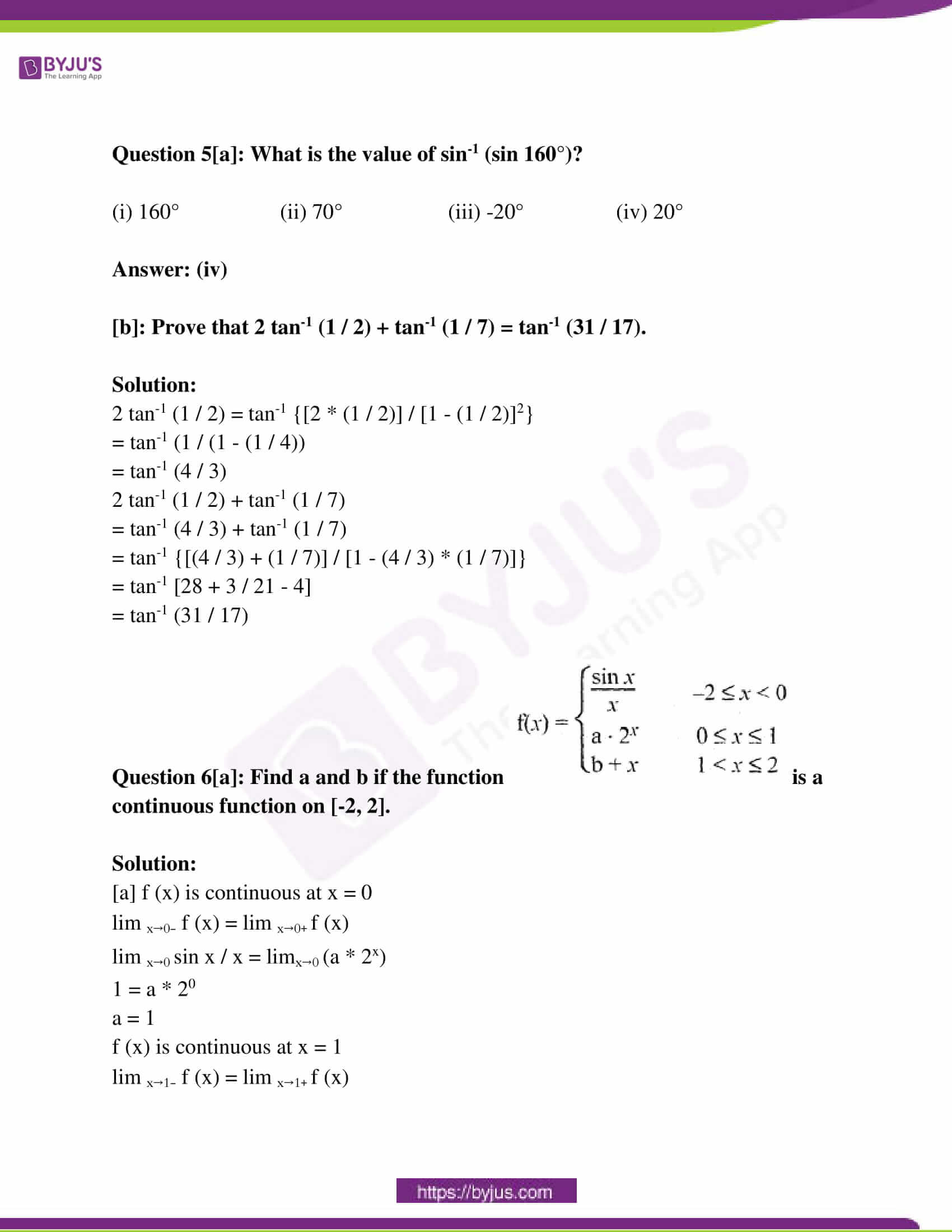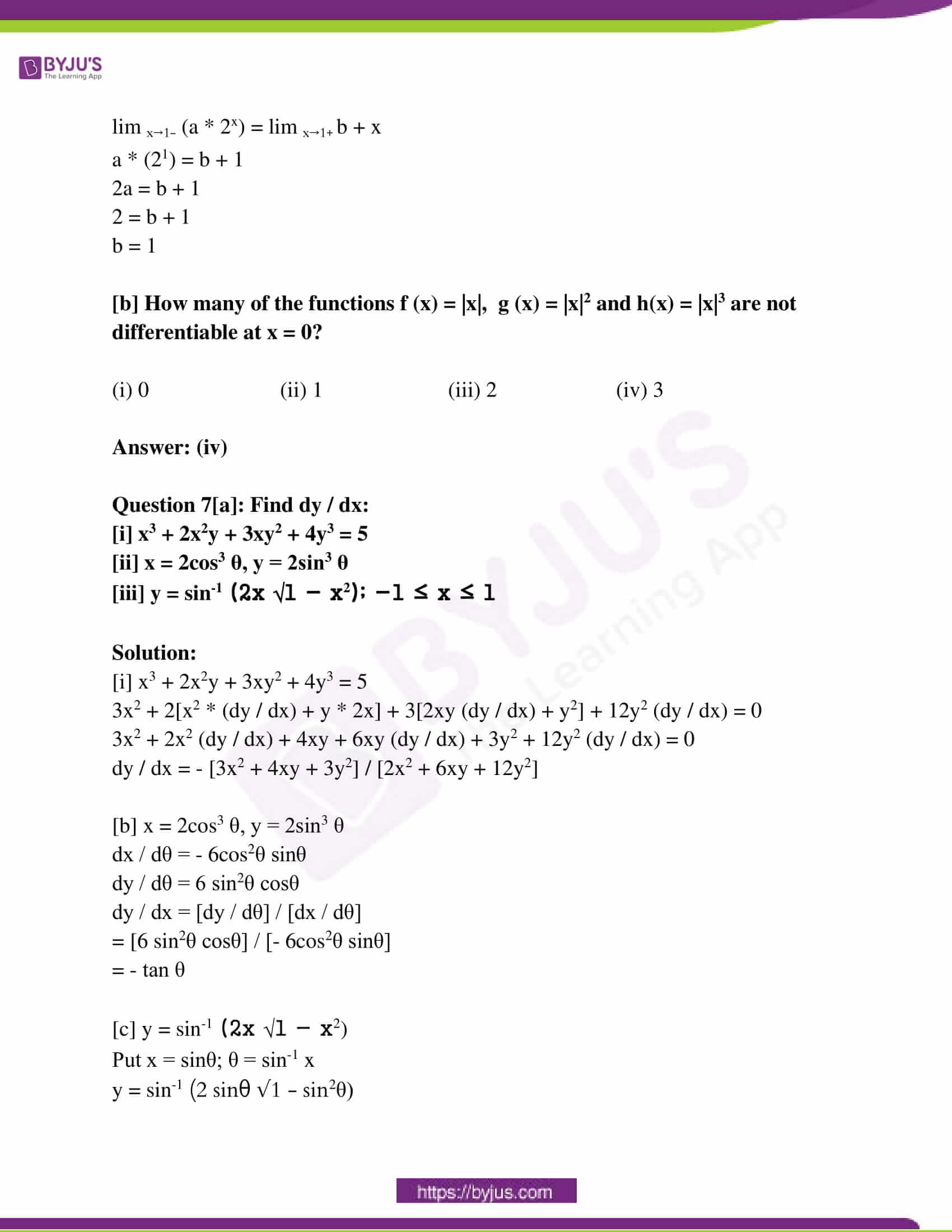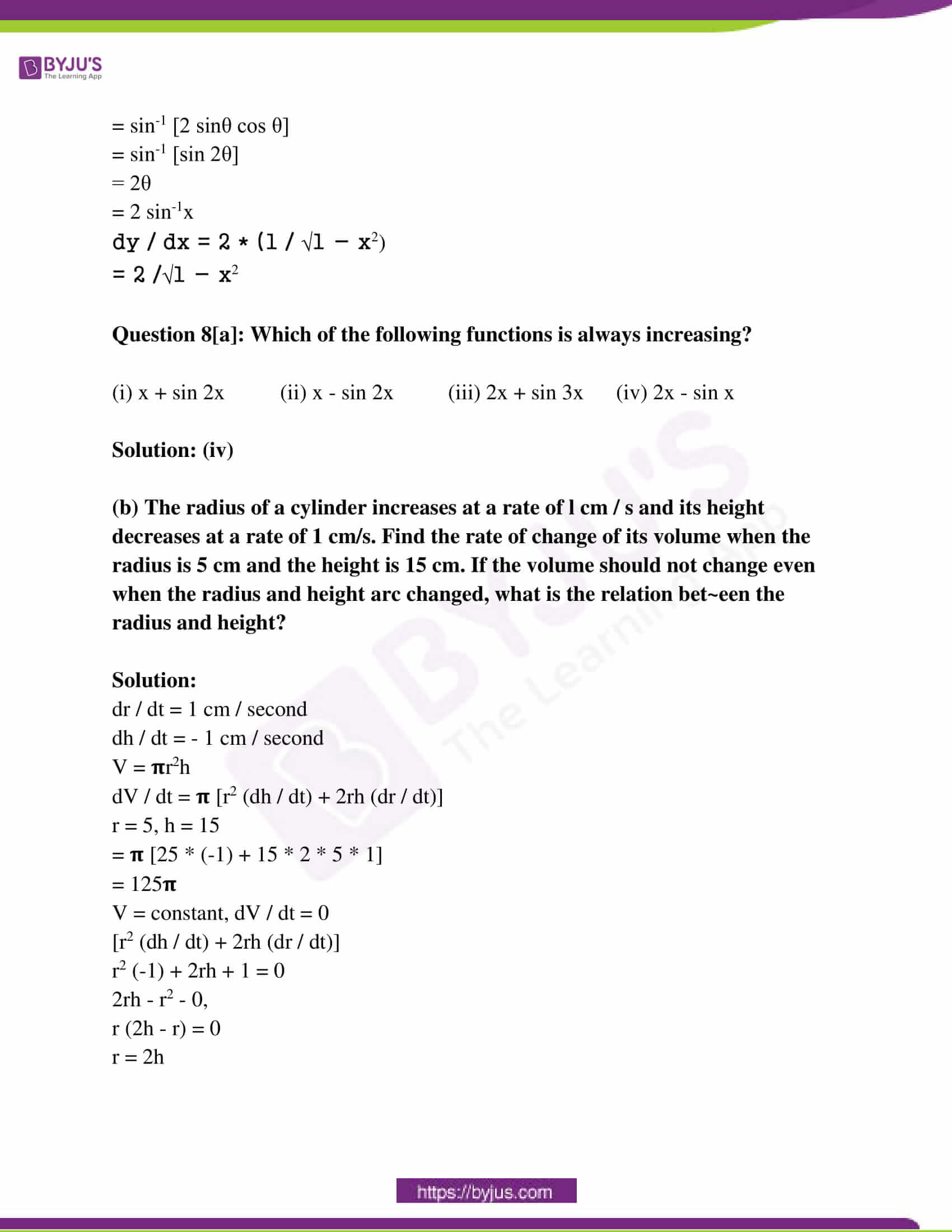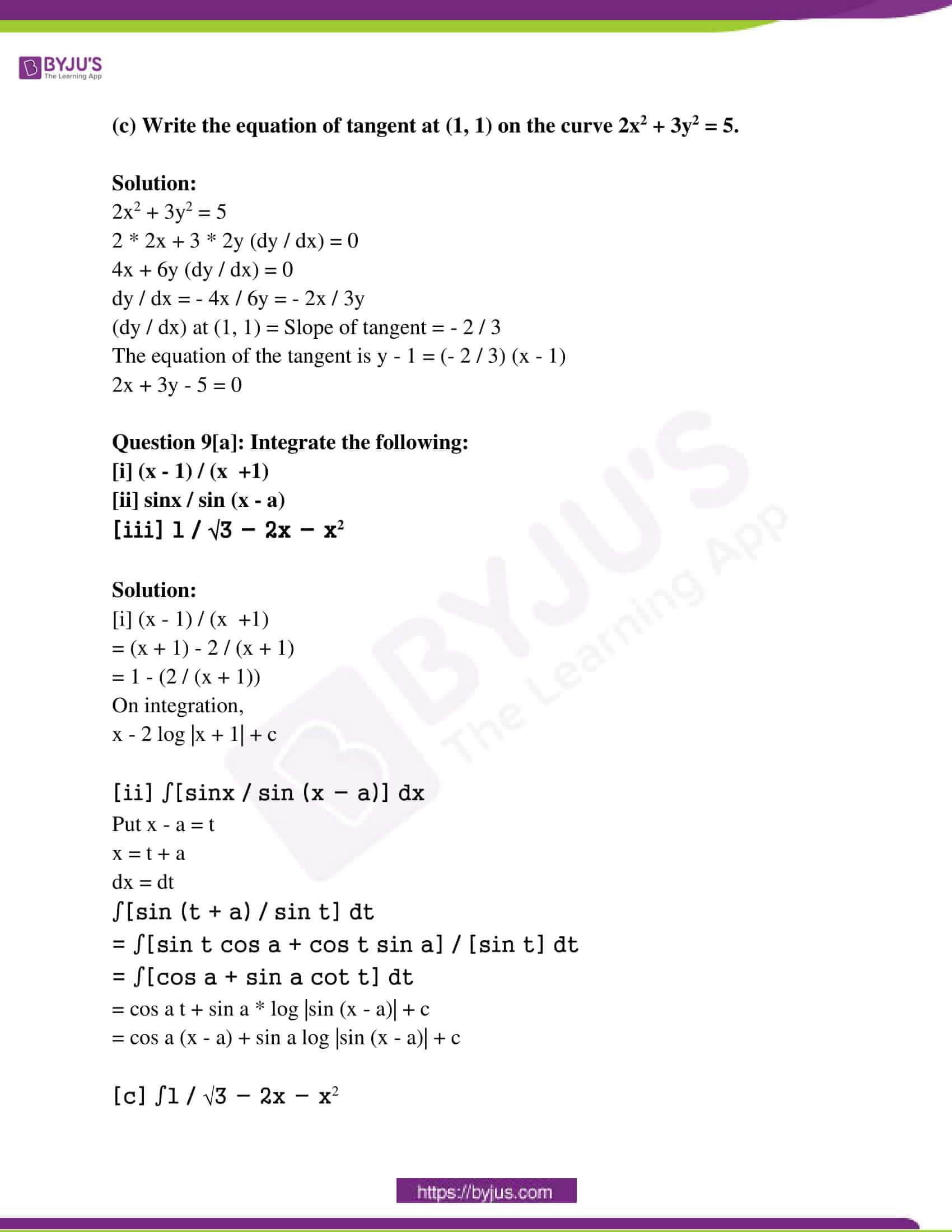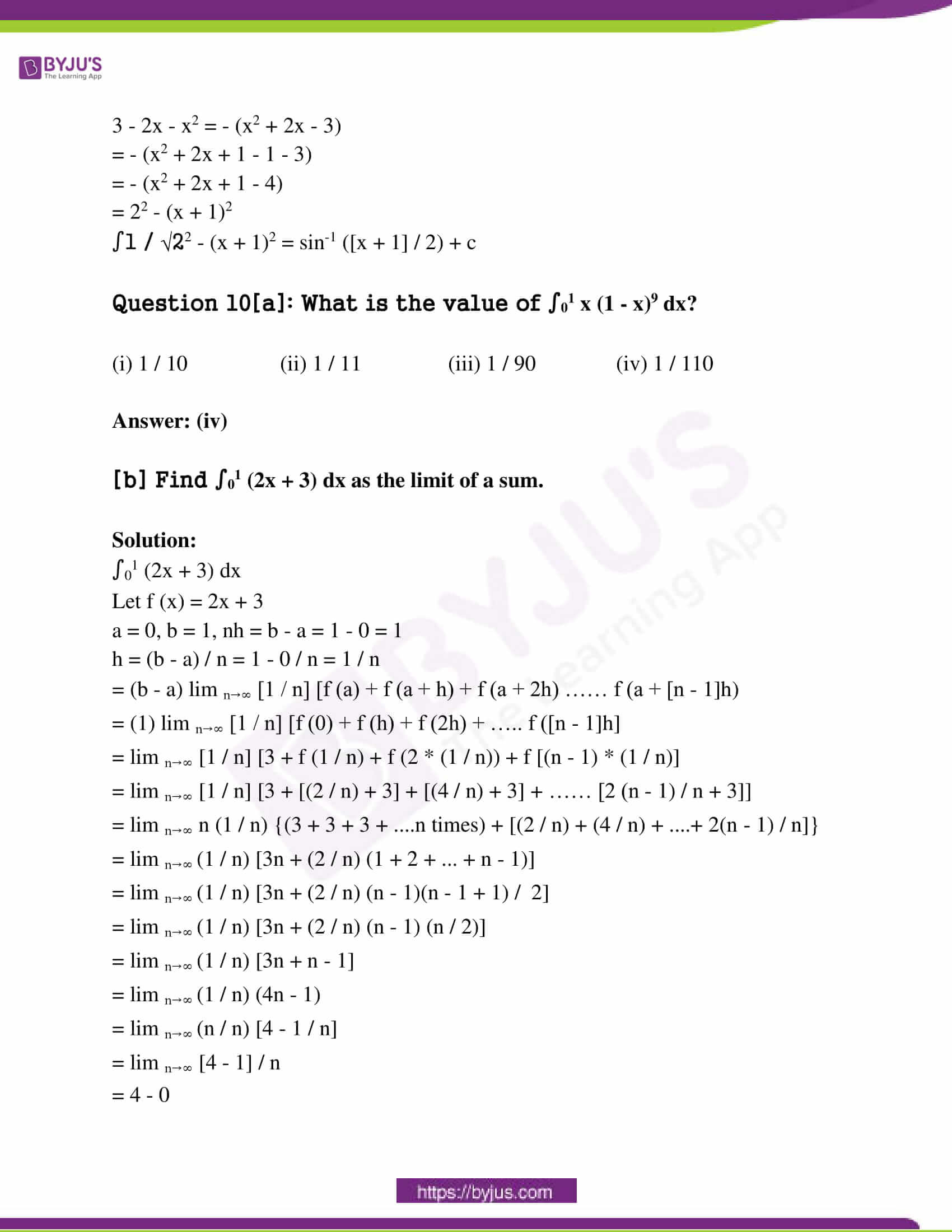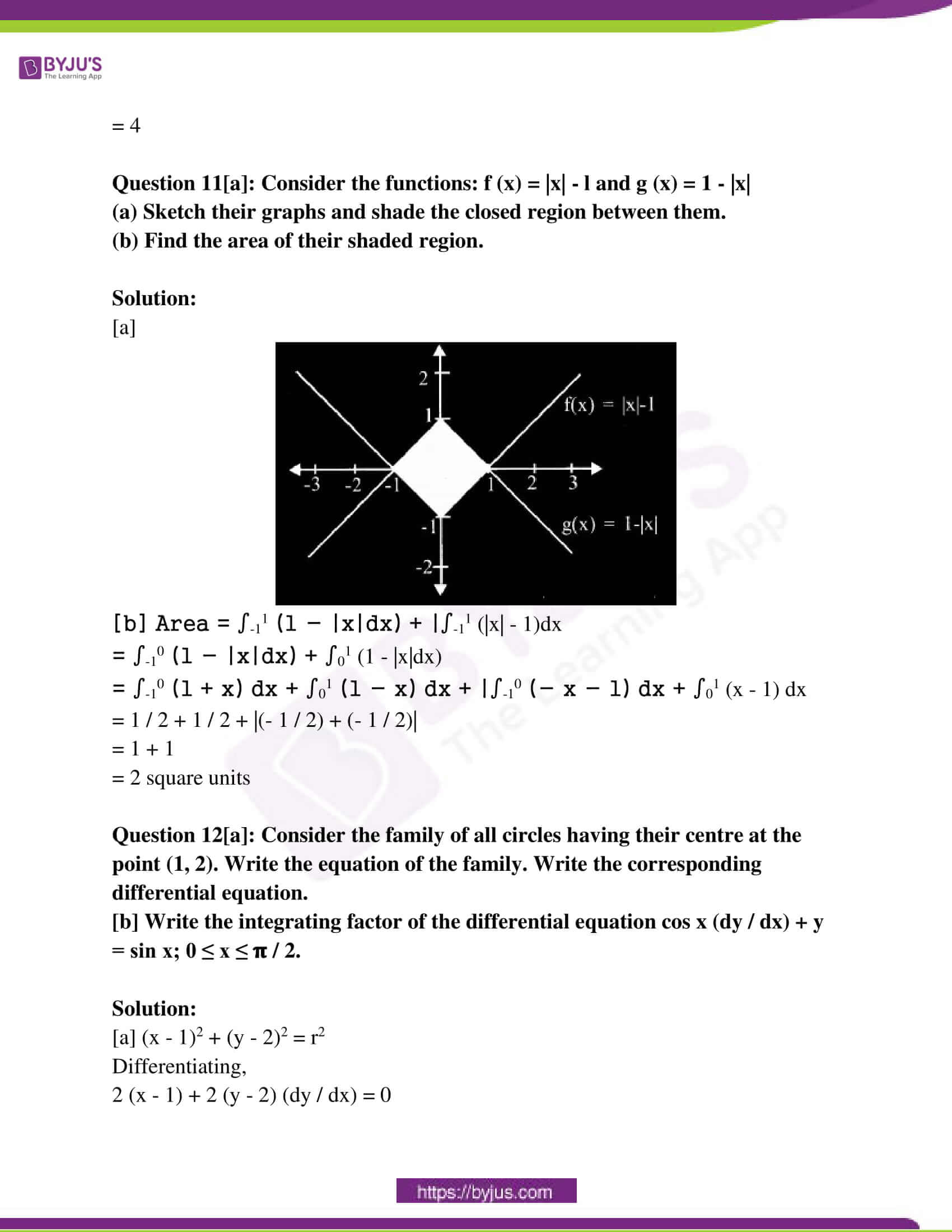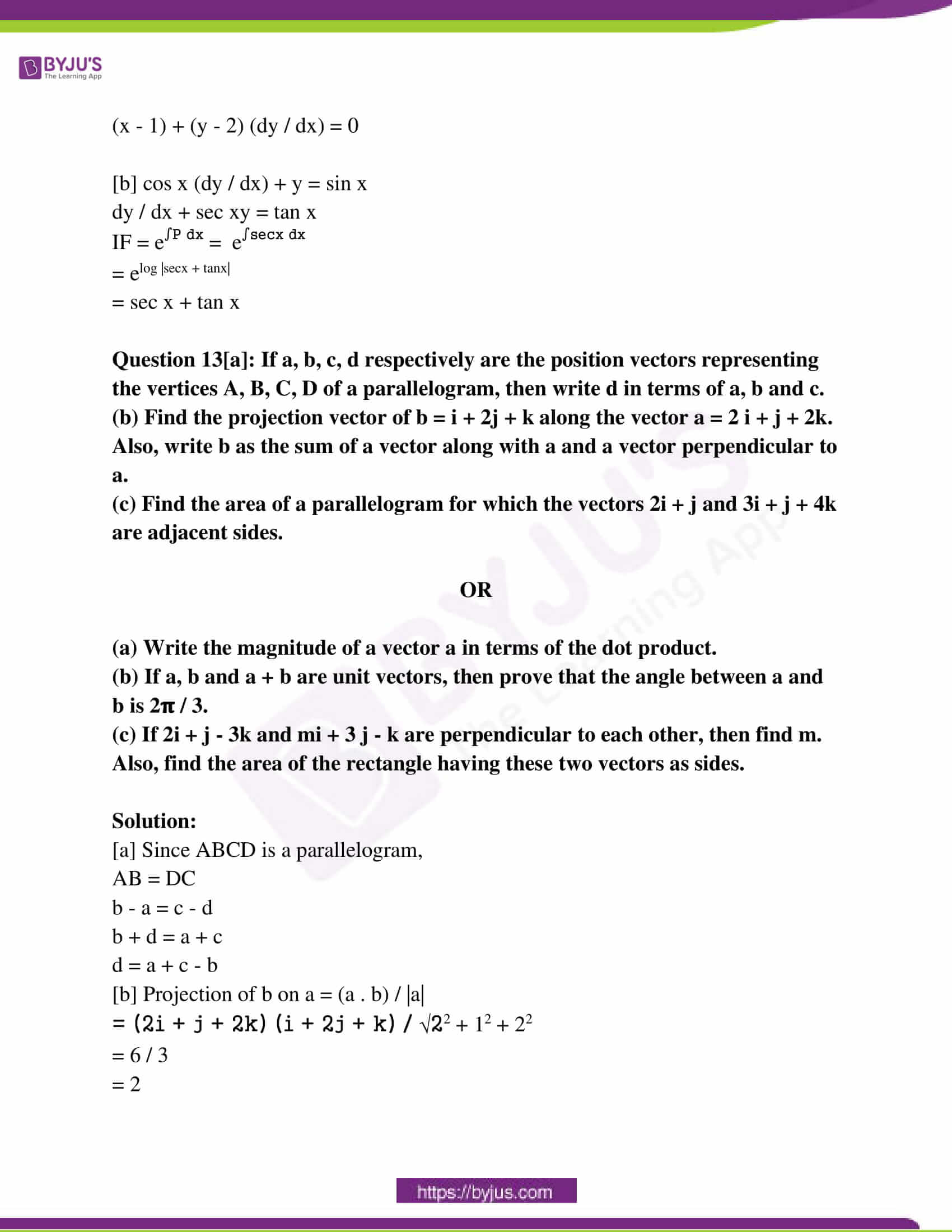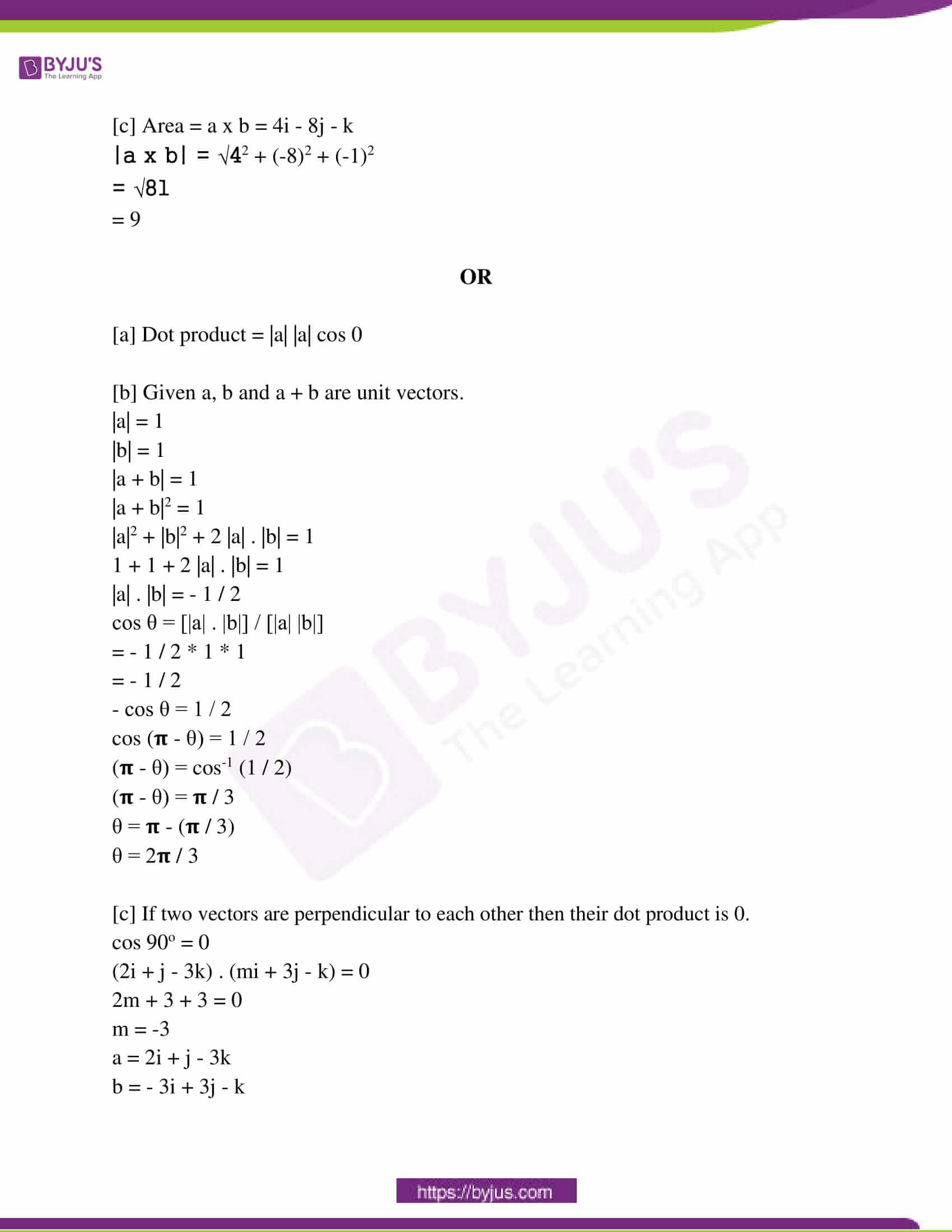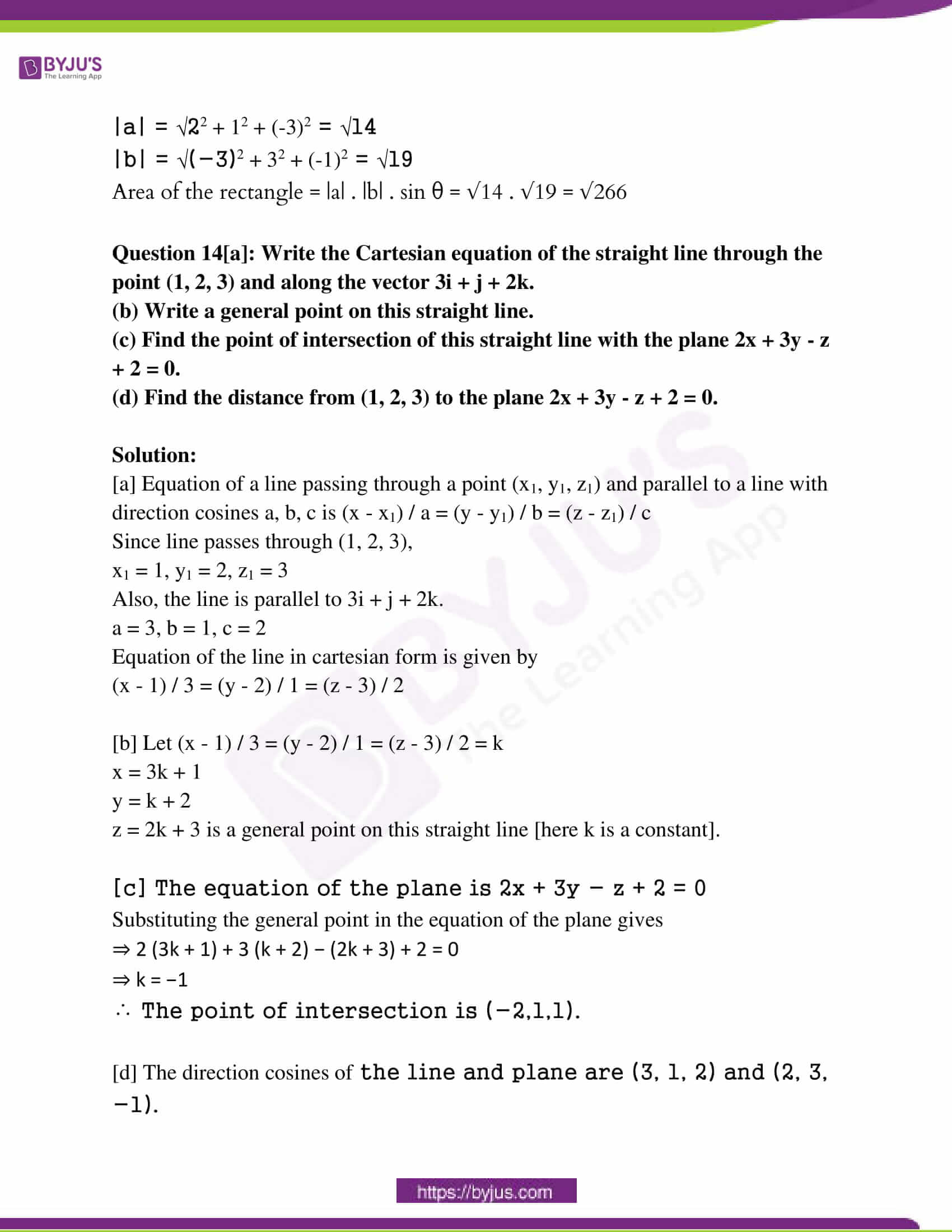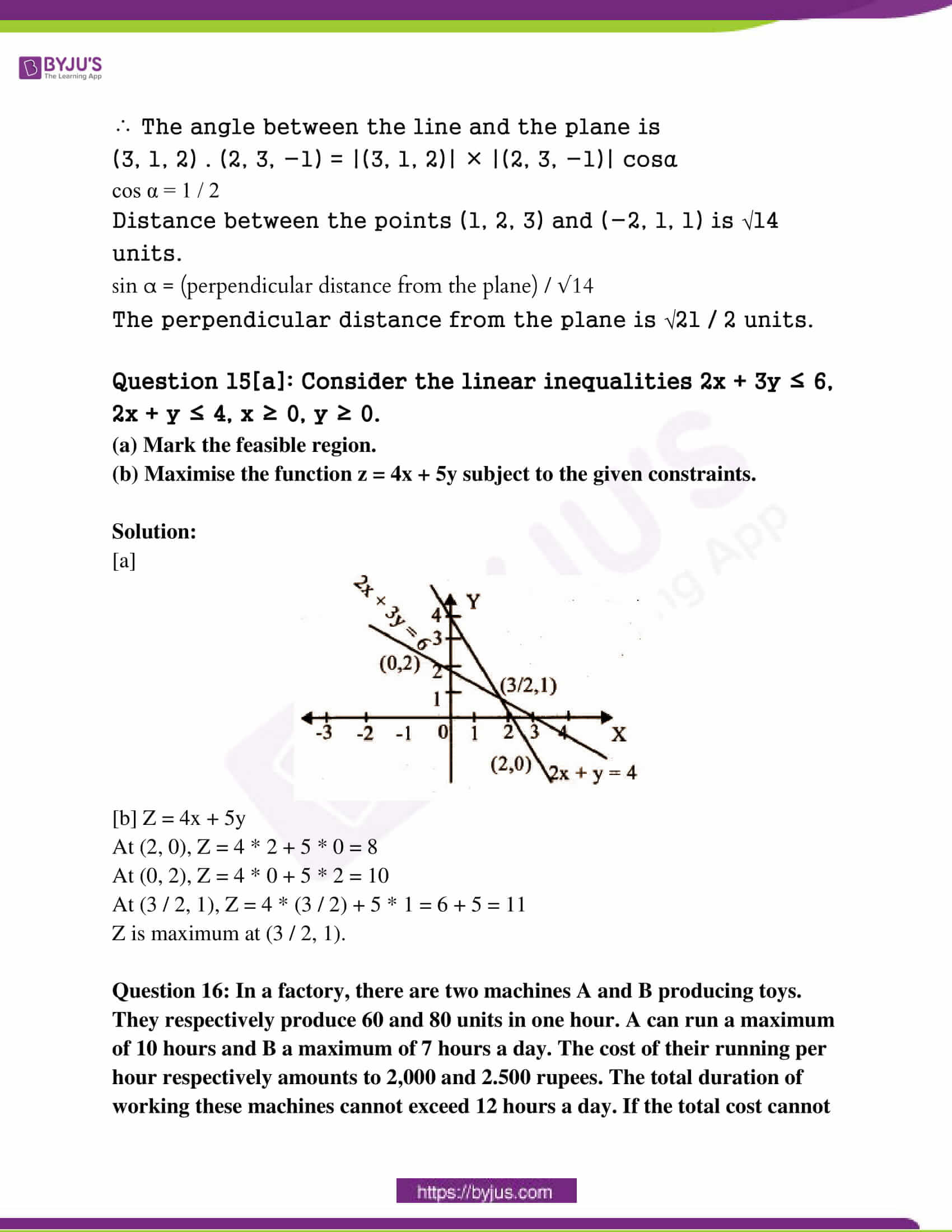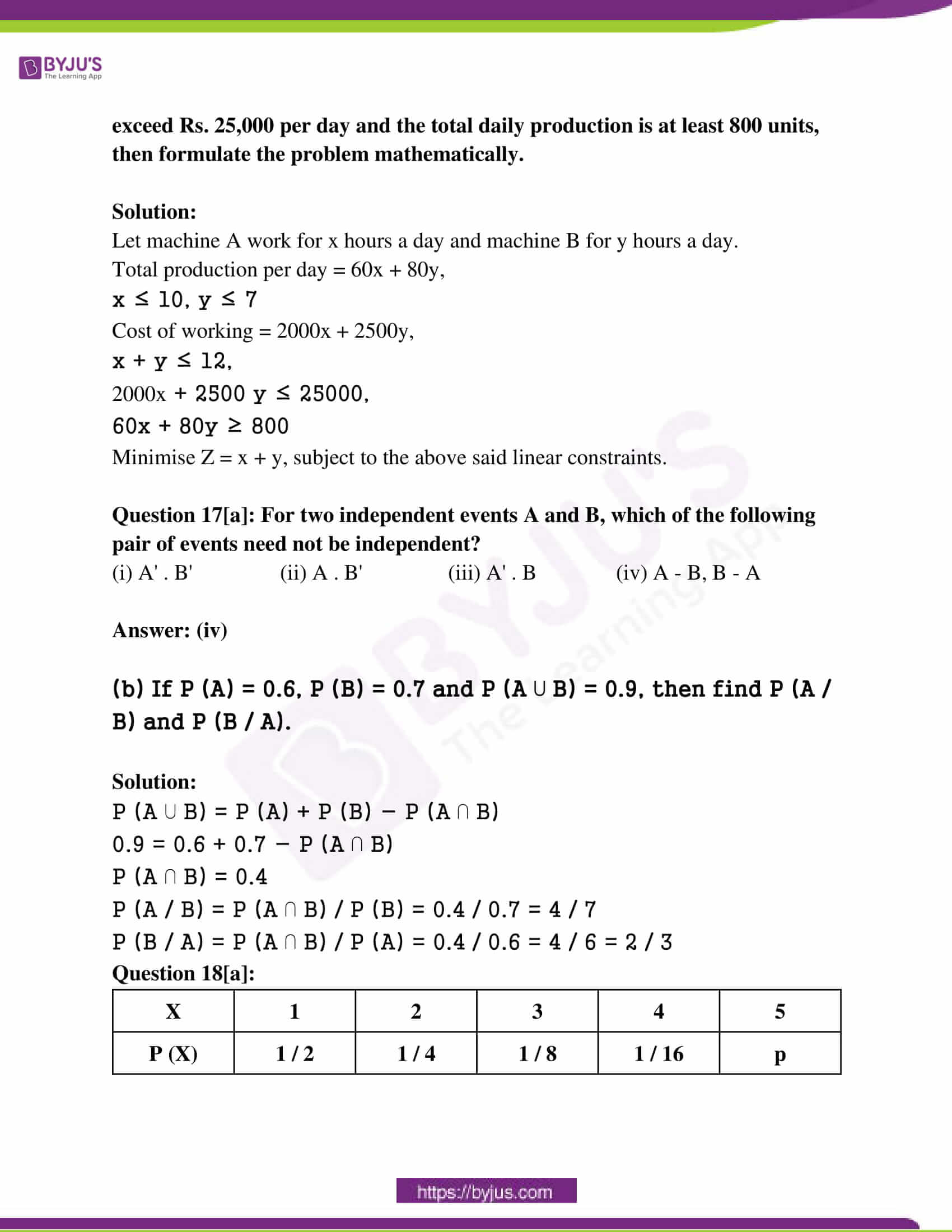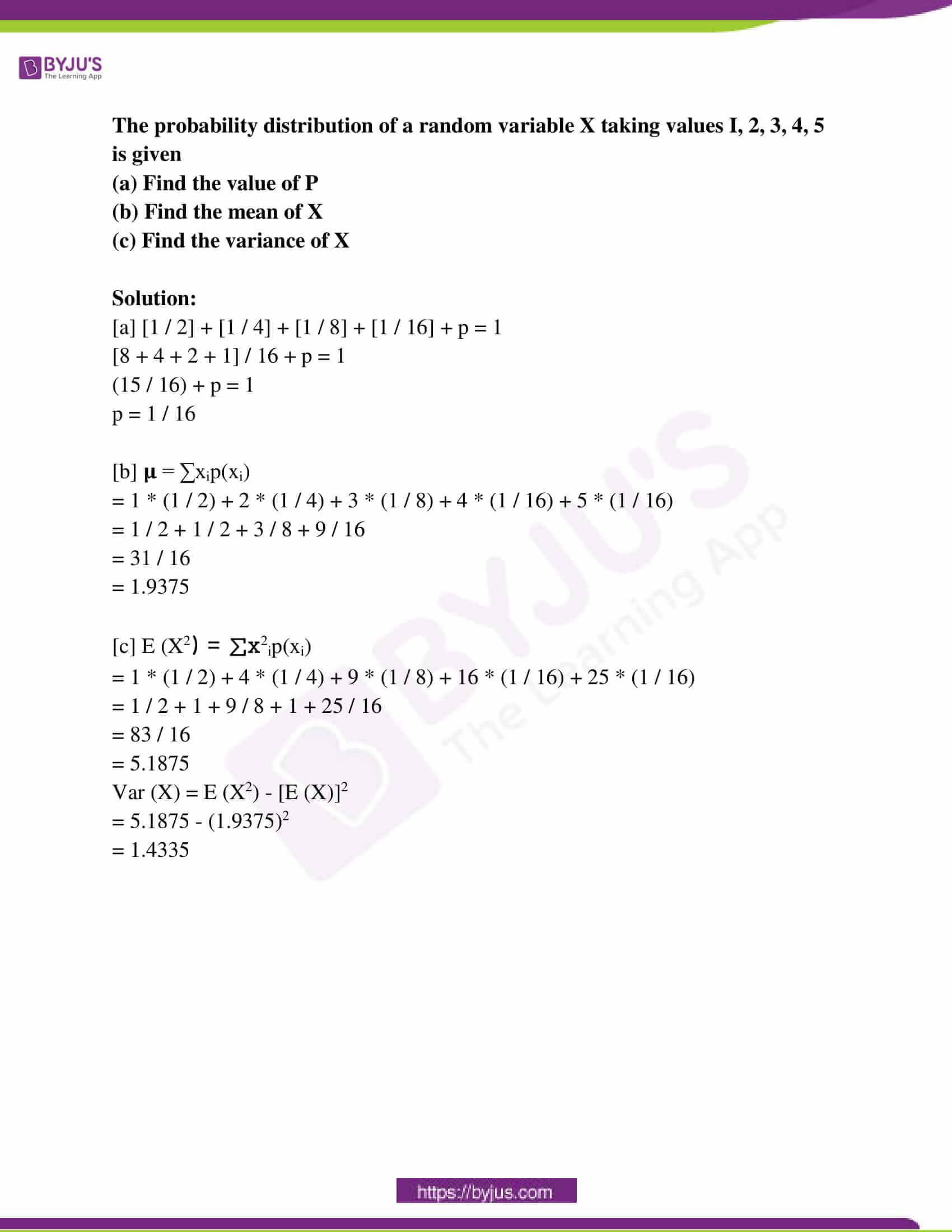Question 1[a]: Choose the correct statement related to matrices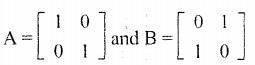(i) A3 = A, B3 ≠ 0 (ii) A3 ≠ A, B3 = B

(iii) A3 = A, B3 = B (iv) A3 ≠ A, B3 ≠ B

[b] If M =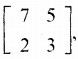then verify the equation M2 – 10M + 11I2 = 0.

Solution: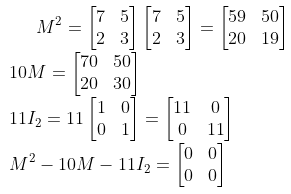[c] Inverse of the matrix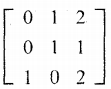.

Solution: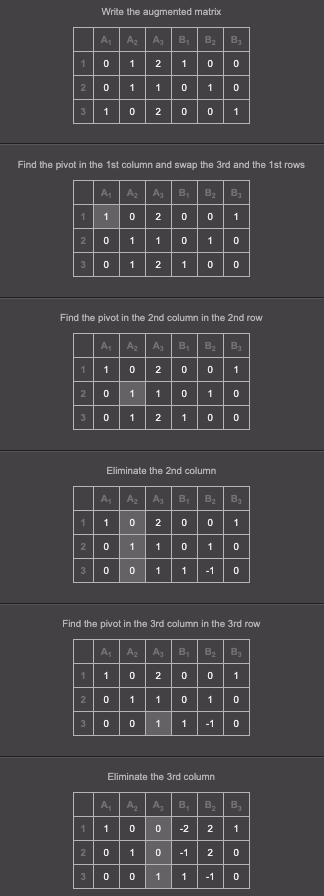There is the inverse matrix on the right.

Question 2[a]: Prove that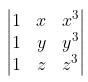= (x + y + z) (x – y) (y – z) (z – x).

OR

[b] Prove that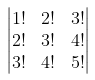= 4!.

Solution:

[a]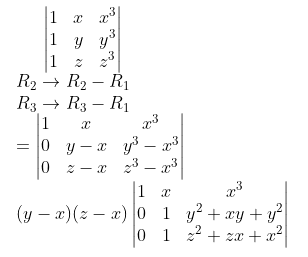= (y – x) (z – x) [(z2 + zx + x2) – (y2 + xy + x2)]

= (y – x) (z – x) [z2 – y2 + x (z – y)]

= (y – x) (z – x) [(z + y) (z – y) + x (z – y)]

= (y – x) (z – x) [(z – y) (x + y + z)]

= (x + y + z) (x – y) (y – z) (z – x)

OR

[b]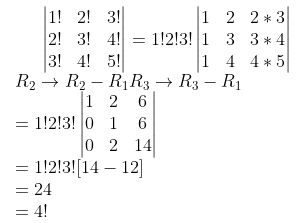Question 3: Solve the system of linear equations.

x + 2y + z = 8

2x + y – z = 1

x – y + z = 2

Solution: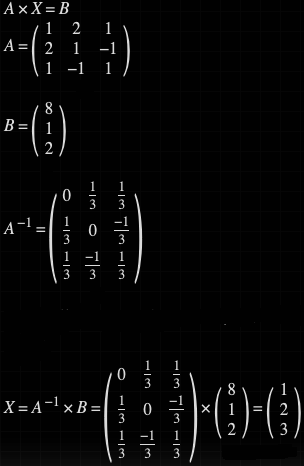Question 4[a]: What is the minimum number of ordered pairs to form a non-zero reflexive relation on a set of n elements?

Solution:

[a] n

[b] On the set R of real numbers, S is a relation defined as S = {(x, y) x ∈ R, y ∈ R, x + y = xy}. Find a ∈ R such that ‘a’ is never the first element of an ordered pair in S. Also find b ∈ R such that ‘b’ is never the second element of an ordered pair in S.

Solution:

R = {(x , y) / x ∈ R, y ∈ R, x + y = xy}

x + y = xy is not defined when x = 1, y = 1.

Hence, a = 1, b =1.

[c] Consider the function f (x) = 3x + 4 / x – 2; x ≠ 2. Find a function g(x) on a suitable domain such that (g o f) (x) = x = (f o g) (x).

Solution:

f (x) = 3x + 4 / x – 2

(g o f) (x) = x = (f o g) (x)

So, g (x) is the inverse of f (x).

y = 3x + 4 / x – 2

y (x – 2) = 3x + 4

xy – 2y = 3x + 4

xy – 3x = 4 + 2y

x (y – 3) = 4 + 2y

x = (4 + 2y) / (y – 3)

f -1 (y) = (4 + 2y) / (y – 3)

f -1 (x) = (4 + 2x) / (x – 3)

Question 5[a]: What is the value of sin-1 (sin 160°)?

(i) 160° (ii) 70° (iii) -20° (iv) 20°

[b]: Prove that 2 tan-1 (1 / 2) + tan-1 (1 / 7) = tan-1 (31 / 17).

Solution:

2 tan-1 (1 / 2) = tan-1 {[2 * (1 / 2)] / [1 – (1 / 2)]2}

= tan-1 (1 / (1 – (1 / 4))

= tan-1 (4 / 3)

2 tan-1 (1 / 2) + tan-1 (1 / 7)

= tan-1 (4 / 3) + tan-1 (1 / 7)

= tan-1 {[(4 / 3) + (1 / 7)] / [1 – (4 / 3) * (1 / 7)]}

= tan-1 [28 + 3 / 21 – 4]

= tan-1 (31 / 17)

Question 6[a]: Find a and b if the function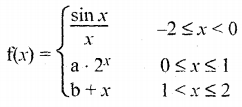is a continuous function on [-2, 2].

Solution:

[a] f (x) is continuous at x = 0

lim x→0- f (x) = lim x→0+ f (x)

lim x→0 sin x / x = limx→0 (a * 2x)

1 = a * 20

a = 1

f (x) is continuous at x = 1

lim x→1- f (x) = lim x→1+ f (x)

lim x→1- (a * 2x) = lim x→1+ b + x

a * (21) = b + 1

2a = b + 1

2 = b + 1

b = 1

[b] How many of the functions f (x) = |x|, g (x) = |x|2 and h(x) = |x|3 are not differentiable at x = 0?

(i) 0 (ii) 1 (iii) 2 (iv) 3

Question 7[a]: Find dy / dx:

[i] x3 + 2x2y + 3xy2 + 4y3 = 5

[ii] x = 2cos3 θ, y = 2sin3 θ

[iii] y = sin-1 (2x √1 – x2); -1 ≤ x ≤ 1

Solution:

[i] x3 + 2x2y + 3xy2 + 4y3 = 5

3x2 + 2[x2 * (dy / dx) + y * 2x] + 3[2xy (dy / dx) + y2] + 12y2 (dy / dx) = 0

3x2 + 2x2 (dy / dx) + 4xy + 6xy (dy / dx) + 3y2 + 12y2 (dy / dx) = 0

dy / dx = – [3x2 + 4xy + 3y2] / [2x2 + 6xy + 12y2]

[b] x = 2cos3 θ, y = 2sin3 θ

dx / dθ = – 6cos2θ sinθ

dy / dθ = 6 sin2θ cosθ

dy / dx = [dy / dθ] / [dx / dθ]

= [6 sin2θ cosθ] / [- 6cos2θ sinθ]

= – tan θ

[c] y = sin-1 (2x √1 – x2)

Put x = sinθ; θ = sin-1 x

y = sin-1 (2 sinθ √1 – sin2θ)

= sin-1 [2 sinθ cos θ]

= sin-1 [sin 2θ]

= 2θ

= 2 sin-1x

dy / dx = 2 * (1 / √1 – x2)

= 2 /√1 – x2

Question 8[a]: Which of the following functions is always increasing?

(i) x + sin 2x (ii) x – sin 2x (iii) 2x + sin 3x (iv) 2x – sin x

Solution: (iv)

(b) The radius of a cylinder increases at a rate of l cm / s and its height decreases at a rate of 1 cm/s. Find the rate of change of its volume when the radius is 5 cm and the height is 15 cm. If the volume should not change even when the radius and height arc changed, what is the relation bet~een the radius and height?

Solution:

dr / dt = 1 cm / second

dh / dt = – 1 cm / second

V = 𝛑r2h

dV / dt = 𝛑 [r2 (dh / dt) + 2rh (dr / dt)]

r = 5, h = 15

= 𝛑 [25 * (-1) + 15 * 2 * 5 * 1]

= 125𝛑

V = constant, dV / dt = 0

[r2 (dh / dt) + 2rh (dr / dt)]

r2 (-1) + 2rh + 1 = 0

2rh – r2 – 0,

r (2h – r) = 0

r = 2h

(c) Write the equation of tangent at (1, 1) on the curve 2x2 + 3y2 = 5.

Solution:

2x2 + 3y2 = 5

2 * 2x + 3 * 2y (dy / dx) = 0

4x + 6y (dy / dx) = 0

dy / dx = – 4x / 6y = – 2x / 3y

(dy / dx) at (1, 1) = Slope of tangent = – 2 / 3

The equation of the tangent is y – 1 = (- 2 / 3) (x – 1)

2x + 3y – 5 = 0

Question 9[a]: Integrate the following:

[i] (x – 1) / (x +1)

[ii] sinx / sin (x – a)

[iii] 1 / √3 – 2x – x2

Solution:

[i] (x – 1) / (x +1)

= (x + 1) – 2 / (x + 1)

= 1 – (2 / (x + 1))

On integration,

x – 2 log |x + 1| + c

[ii] ∫[sinx / sin (x – a)] dx

Put x – a = t

x = t + a

dx = dt

∫[sin (t + a) / sin t] dt

= ∫[sin t cos a + cos t sin a] / [sin t] dt

= ∫[cos a + sin a cot t] dt

= cos a t + sin a * log |sin (x – a)| + c

= cos a (x – a) + sin a log |sin (x – a)| + c

[c] ∫1 / √3 – 2x – x2

3 – 2x – x2 = – (x2 + 2x – 3)

= – (x2 + 2x + 1 – 1 – 3)

= – (x2 + 2x + 1 – 4)

= 22 – (x + 1)2

∫1 / √22 – (x + 1)2 = sin-1 ([x + 1] / 2) + c

Question 10[a]: What is the value of ∫01 x (1 – x)9 dx?

(i) 1 / 10 (ii) 1 / 11 (iii) 1 / 90 (iv) 1 / 110

[b] Find ∫01 (2x + 3) dx as the limit of a sum.

Solution:

01 (2x + 3) dx

Let f (x) = 2x + 3

a = 0, b = 1, nh = b – a = 1 – 0 = 1

h = (b – a) / n = 1 – 0 / n = 1 / n

= (b – a) lim n→∞ [1 / n] [f (a) + f (a + h) + f (a + 2h) …… f (a + [n – 1]h)

= (1) lim n→∞ [1 / n] [f (0) + f (h) + f (2h) + ….. f ([n – 1]h]

= lim n→∞ [1 / n] [3 + f (1 / n) + f (2 * (1 / n)) + f [(n – 1) * (1 / n)]

= lim n→∞ [1 / n] [3 + [(2 / n) + 3] + [(4 / n) + 3] + …… [2 (n – 1) / n + 3]]

= lim n→∞ n (1 / n) {(3 + 3 + 3 + ….n times) + [(2 / n) + (4 / n) + ….+ 2(n – 1) / n]}

= lim n→∞ (1 / n) [3n + (2 / n) (1 + 2 + … + n – 1)]

= lim n→∞ (1 / n) [3n + (2 / n) (n – 1)(n – 1 + 1) / 2]

= lim n→∞ (1 / n) [3n + (2 / n) (n – 1) (n / 2)]

= lim n→∞ (1 / n) [3n + n – 1]

= lim n→∞ (1 / n) (4n – 1)

= lim n→∞ (n / n) [4 – 1 / n]

= lim n→∞ [4 – 1] / n

= 4 – 0

= 4

Question 11[a]: Consider the functions: f (x) = |x| – l and g (x) = 1 – |x|

(a) Sketch their graphs and shade the closed region between them.

(b) Find the area of their shaded region.

Solution:

[a]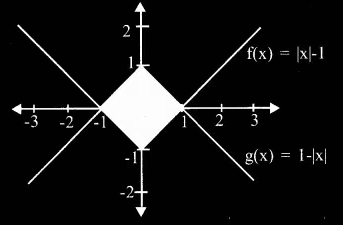[b] Area = ∫-11 (1 – |x|dx) + |∫-11 (|x| – 1)dx

= ∫-10 (1 – |x|dx) + ∫01 (1 – |x|dx)

= ∫-10 (1 + x) dx + ∫01 (1 – x) dx + |∫-10 (- x – 1) dx + ∫01 (x – 1) dx

= 1 / 2 + 1 / 2 + |(- 1 / 2) + (- 1 / 2)|

= 1 + 1

= 2 square units

Question 12[a]: Consider the family of all circles having their centre at the point (1, 2). Write the equation of the family. Write the corresponding differential equation.

[b] Write the integrating factor of the differential equation cos x (dy / dx) + y = sin x; 0 ≤ x ≤ 𝛑 / 2.

Solution:

[a] (x – 1)2 + (y – 2)2 = r2

Differentiating,

2 (x – 1) + 2 (y – 2) (dy / dx) = 0

(x – 1) + (y – 2) (dy / dx) = 0

[b] cos x (dy / dx) + y = sin x

dy / dx + sec xy = tan x

IF = e∫P dx = e∫secx dx

= elog |secx + tanx|

= sec x + tan x

Question 13[a]: If a, b, c, d respectively are the position vectors representing the vertices A, B, C, D of a parallelogram, then write d in terms of a, b and c.

(b) Find the projection vector of b = i + 2j + k along the vector a = 2 i + j + 2k. Also, write b as the sum of a vector along with a and a vector perpendicular to a.

(c) Find the area of a parallelogram for which the vectors 2i + j and 3i + j + 4k are adjacent sides.

OR

(a) Write the magnitude of a vector a in terms of the dot product.

(b) If a, b and a + b are unit vectors, then prove that the angle between a and b is 2𝛑 / 3.

(c) If 2i + j – 3k and mi + 3 j – k are perpendicular to each other, then find m. Also, find the area of the rectangle having these two vectors as sides.

Solution:

[a] Since ABCD is a parallelogram,

AB = DC

b – a = c – d

b + d = a + c

d = a + c – b

[b] Projection of b on a = (a . b) / |a|

= (2i + j + 2k) (i + 2j + k) / √22 + 12 + 22

= 6 / 3

= 2

[c] Area = a x b = 4i – 8j – k

|a x b| = √42 + (-8)2 + (-1)2

= √81

= 9

OR

[a] Dot product = |a| |a| cos 0

[b] Given a, b and a + b are unit vectors.

|a| = 1

|b| = 1

|a + b| = 1

|a + b|2 = 1

|a|2 + |b|2 + 2 |a| . |b| = 1

1 + 1 + 2 |a| . |b| = 1

|a| . |b| = – 1 / 2

cos θ = [|a| . |b|] / [|a| |b|]

= – 1 / 2 * 1 * 1

= – 1 / 2

– cos θ = 1 / 2

cos (𝛑 – θ) = 1 / 2

(𝛑 – θ) = cos-1 (1 / 2)

(𝛑 – θ) = 𝛑 / 3

θ = 𝛑 – (𝛑 / 3)

θ = 2𝛑 / 3

[c] If two vectors are perpendicular to each other then their dot product is 0.

cos 90o = 0

(2i + j – 3k) . (mi + 3j – k) = 0

2m + 3 + 3 = 0

m = -3

a = 2i + j – 3k

b = – 3i + 3j – k

|a| = √22 + 12 + (-3)2 = √14

|b| = √(-3)2 + 32 + (-1)2 = √19

Area of the rectangle = |a| . |b| . sin θ = √14 . √19 = √266

Question 14[a]: Write the Cartesian equation of the straight line through the point (1, 2, 3) and along the vector 3i + j + 2k.

(b) Write a general point on this straight line.

(c) Find the point of intersection of this straight line with the plane 2x + 3y – z + 2 = 0.

(d) Find the distance from (1, 2, 3) to the plane 2x + 3y – z + 2 = 0.

Solution:

[a] Equation of a line passing through a point (x1, y1, z1) and parallel to a line with direction cosines a, b, c is (x – x1) / a = (y – y1) / b = (z – z1) / c

Since line passes through (1, 2, 3),

x1 = 1, y1 = 2, z1 = 3

Also, the line is parallel to 3i + j + 2k.

a = 3, b = 1, c = 2

Equation of the line in cartesian form is given by

(x – 1) / 3 = (y – 2) / 1 = (z – 3) / 2

[b] Let (x – 1) / 3 = (y – 2) / 1 = (z – 3) / 2 = k

x = 3k + 1

y = k + 2

z = 2k + 3 is a general point on this straight line [here k is a constant].

[c] The equation of the plane is 2x + 3y − z + 2 = 0

Substituting the general point in the equation of the plane gives

⇒ 2 (3k + 1) + 3 (k + 2) − (2k + 3) + 2 = 0

⇒ k = −1

∴ The point of intersection is (−2,1,1).

[d] The direction cosines of the line and plane are (3, 1, 2) and (2, 3, −1).

∴ The angle between the line and the plane is

(3, 1, 2) . (2, 3, −1) = |(3, 1, 2)| × |(2, 3, −1)| cosα

cos α = 1 / 2

Distance between the points (1, 2, 3) and (−2, 1, 1) is √14 units.

sin α = (perpendicular distance from the plane) / √14

The perpendicular distance from the plane is √21 / 2 units.

Question 15[a]: Consider the linear inequalities 2x + 3y ≤ 6, 2x + y ≤ 4, x ≥ 0, y ≥ 0.

(a) Mark the feasible region.

(b) Maximise the function z = 4x + 5y subject to the given constraints.

Solution:

[a]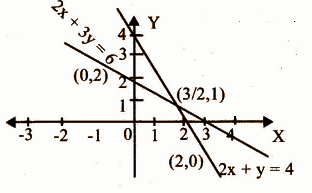[b] Z = 4x + 5y

At (2, 0), Z = 4 * 2 + 5 * 0 = 8

At (0, 2), Z = 4 * 0 + 5 * 2 = 10

At (3 / 2, 1), Z = 4 * (3 / 2) + 5 * 1 = 6 + 5 = 11

Z is maximum at (3 / 2, 1).

Question 16: In a factory, there are two machines A and B producing toys. They respectively produce 60 and 80 units in one hour. A can run a maximum of 10 hours and B a maximum of 7 hours a day. The cost of their running per hour respectively amounts to 2,000 and 2.500 rupees. The total duration of working these machines cannot exceed 12 hours a day. If the total cost cannot exceed Rs. 25,000 per day and the total daily production is at least 800 units, then formulate the problem mathematically.

Solution:

Let machine A work for x hours a day and machine B for y hours a day.

Total production per day = 60x + 80y,

x ≤ 10, y ≤ 7

Cost of working = 2000x + 2500y,

x + y ≤ 12,

2000x + 2500 y ≤ 25000,

60x + 80y ≥ 800

Minimise Z = x + y, subject to the above said linear constraints.

Question 17[a]: For two independent events A and B, which of the following pair of events need not be independent?

(i) A’ . B’ (ii) A . B’ (iii) A’ . B (iv) A – B, B – A

(b) If P (A) = 0.6, P (B) = 0.7 and P (A ⋃ B) = 0.9, then find P (A / B) and P (B / A).

Solution:

P (A ⋃ B) = P (A) + P (B) – P (A ⋂ B)

0.9 = 0.6 + 0.7 – P (A ⋂ B)

P (A ⋂ B) = 0.4

P (A / B) = P (A ⋂ B) / P (B) = 0.4 / 0.7 = 4 / 7

P (B / A) = P (A ⋂ B) / P (A) = 0.4 / 0.6 = 4 / 6 = 2 / 3

Question 18[a]:

 X 1 2 3 4 5 P (X) 1 / 2 1 / 4 1 / 8 1 / 16 p

The probability distribution of a random variable X taking values I, 2, 3, 4, 5 is given

(a) Find the value of P

(b) Find the mean of X

(c) Find the variance of X

Solution:

[a] [1 / 2] + [1 / 4] + [1 / 8] + [1 / 16] + p = 1

[8 + 4 + 2 + 1] / 16 + p = 1

(15 / 16) + p = 1

p = 1 / 16

[b] 𝛍 = ∑xip(xi)

= 1 * (1 / 2) + 2 * (1 / 4) + 3 * (1 / 8) + 4 * (1 / 16) + 5 * (1 / 16)

= 1 / 2 + 1 / 2 + 3 / 8 + 9 / 16

= 31 / 16

= 1.9375

[c] E (X2) = ∑x2ip(xi)

= 1 * (1 / 2) + 4 * (1 / 4) + 9 * (1 / 8) + 16 * (1 / 16) + 25 * (1 / 16)

= 1 / 2 + 1 + 9 / 8 + 1 + 25 / 16

= 83 / 16

= 5.1875

Var (X) = E (X2) – [E (X)]2

= 5.1875 – (1.9375)2

= 1.4335